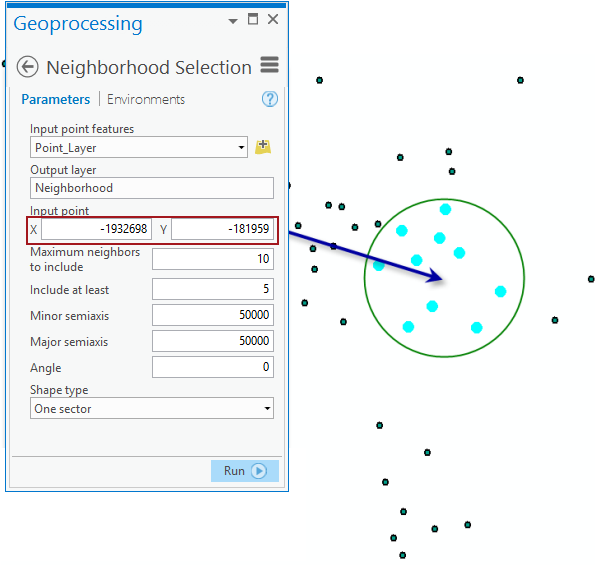# Neighborhood Selection (Geostatistical Analyst)

Geostatistical Analyst のライセンスで利用可能。

## サマリー

Creates a layer of points based on a user-defined neighborhood.The output layer contains the selection set of 10 selected blue points.

For example, you might wish to create a selection of points in a circular neighborhood around a location defined by the Input point. The illustration above demonstrates that the output will be the 10 points, colored blue, that fall within the circle.

## パラメーター

 ラベル 説明 データ タイプ Input point features Points used to create a neighborhood selection. Feature Layer Output layer Layer to store the neighborhood selection. Feature Layer Input point The neighborhood center's x,y coordinate. Point Maximum neighbors to include The number of points to use in each sector. If a sector has the required number of points, all points in that sector are used. Long Include at least The minimum number of points to use in each sector. If the minimum number of required points are not available in any given sector, the nearest available point outside the sector will be selected. Long Minor semiaxis Size of the minor semiaxis of the search neighborhood. Double Major semiaxis Size of the major semiaxis of the search neighborhood. Double Angle The angle of rotation of the neighborhood axis. Double Shape type(オプション) The geometry of the neighborhood.One sector— Single ellipseFour sectors— Ellipse divided into four sectorsFour shifted sectors— Ellipse divided into four sectors and shifted 45 degreesEight sectors— Ellipse divided into eight sectors String

`arcpy.ga.GANeighborhoodSelection(in_dataset, out_layer, point_coord, neighbors_max, neighbors_min, minor_semiaxis, major_semiaxis, angle, {shape_type})`
 名前 説明 データ タイプ in_dataset Points used to create a neighborhood selection. Feature Layer out_layer Layer to store the neighborhood selection. Feature Layer point_coord The neighborhood center's x,y coordinate. Point neighbors_max The number of points to use in each sector. If a sector has the required number of points, all points in that sector are used. Long neighbors_min The minimum number of points to use in each sector. If the minimum number of required points are not available in any given sector, the nearest available point outside the sector will be selected. Long minor_semiaxis Size of the minor semiaxis of the search neighborhood. Double major_semiaxis Size of the major semiaxis of the search neighborhood. Double angle The angle of rotation of the neighborhood axis. Double shape_type(オプション) The geometry of the neighborhood.ONE_SECTOR— Single ellipseFOUR_SECTORS— Ellipse divided into four sectorsFOUR_SECTORS_SHIFTED— Ellipse divided into four sectors and shifted 45 degreesEIGHT_SECTORS— Ellipse divided into eight sectors String

### コードのサンプル

NeighborhoodSelection example 1 (Python window)

Create a feature layer based on some neighborhood criteria.

``````import arcpy
from arcpy import env
env.workspace = "C:/gapyexamples/data"
arcpy.GANeighborhoodSelection_ga("ca_ozone_pts", "outNS", "-2000000 -50000",
"20", "5","200000", "200000", "0", "One sector")``````
NeighborhoodSelection example 2 (stand-alone script)

Create a feature layer based on some neighborhood criteria.

``````# Name: NeighborhoodSelection_Example_02.py
# Description: Creates a layer of points based on a user-defined neighborhood.
# Requirements: Geostatistical Analyst Extension

# Import system modules
import arcpy

# Set environment settings
arcpy.env.workspace = "C:/gapyexamples/data"

# Set local variables
inPoints = "ca_ozone_pts.shp"
outLayer = "outNS"
pointCoord = "-2000000 -50000"
maxNeighbors = 20
minNeighbors = 5
majSemiaxis = 200000
minSemiaxis = 200000
angle = 0
shape = "One Sector"

# Execute NeighborhoodSelection
arcpy.GANeighborhoodSelection_ga(inPoints, outLayer, pointCoord, maxNeighbors,
minNeighbors, majSemiaxis, minSemiaxis, angle,
shape)``````

## ライセンス情報

• Basic: 次のものが必要 Geostatistical Analyst
• Standard: 次のものが必要 Geostatistical Analyst